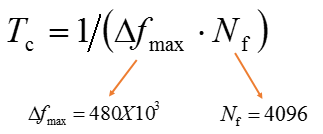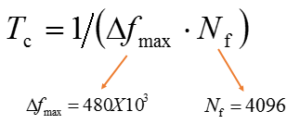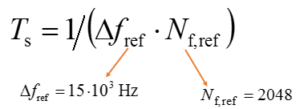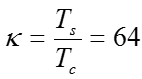5g technology

# 5G NR- Physical Layer Timing Unit## 5G NR- Physical Layer Timing Unit

In 5 G New Radio the basic time unit is represented by Tand as per 3GPP TS38.211 can be expression by following equation:Where is subcarrier spacing and is FFT size. Considering above  equation timing can be calculated as follows:
T= 1 / (480 x 103  x 4096)

= 1 / 1966080000
= 1 x 10 -3 / 196608 = 5.0862 x 10-10
= 0.509 nano second

LTE basic time unit is represented by Ts and expressed by following equationTs =  1 / (15 x 103  x 2048)
= 1 / 30720000
=  1 x 10 -3 / 30720 = 3.2552 x 10-8
= 32.55 nano second

Ts and  Tc  Relation
The specification provided a constantto relate NR basic time unit and LTE basic time unit and represented by following equation.